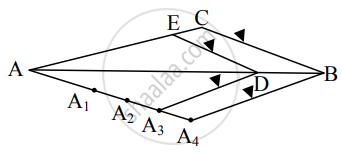# Choose the correct alternative: In the figure ΔABC ~ ΔADE then the ratio of their corresponding sides is - Geometry

MCQ
Fill in the Blanks

Choose the correct alternative:In the figure ΔABC ~ ΔADE then the ratio of their corresponding sides is ______

• 3/1

• 1/3

• 3/4

• 4/3

#### Solution

4/3From the given figure, we get that

AD = 3 units, DB = 1 units, and AB = 4 units   ...(i)

As ∆ABC ∼ ∆ADE, we get

"AB"/"AD" = "BC"/"DE" = "AC"/"AE"     ...[Ratio of Corresponding sides of similar triangles]

∴ 4/3 = "BC"/"DE" = "AC"/"AE"          ...[From (i)]

Concept: Division of a Line Segment
Is there an error in this question or solution?